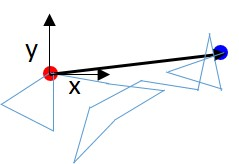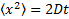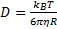# Gradients in NMR Spectroscopy – Part 4: A short Intermezzo on Diffusion

Part 3 discussed how a matched pair of positive/negative magnetic field gradient pulses can be used to encode spins for their displacement. We can distinguish between two basic types of displacement:

• All spins are moving together with the same speed along the same direction. This leads to a phase shift in the signal.
• All spins are jumping randomly between positions. This results in an attenuation of the signal amplitude.

In this post we will have a closer look at this second type of movement.

It is closely related to the spreading of for example a blob of ink in a beaker filled with water, which is shown schematically in Figure 1. Initially, the blob is located within a small volume (a) near the wall, but over time it will spread out and eventually cover the entire volume (b). The Latin word for “spreading out” is “diffundere”, and therefore this process is known as diffusion.Figure 1: (a) Some particles are dissolved in a beaker of water. Initially, the particles are located in a small volume near the wall. Over time, the particles randomly move around and spread evenly over the entire volume (b).

This type of random jumps was first visually observed by the Scottish botanist Robert Brown when he watched pollen grains suspended in water. Nowadays we know that diffusion (another name is Brownian motion) governs biochemical processes, and there is a great interest to measure and understand such random movements.

Let us look at the path of a single particle undergoing a random movement. Let’s assume that the movement is not restricted by walls or other particles along the way. Each jump will be of the same length, but their directions will be evenly distributed. Then the path in two dimensions will look similar to the one shown in Figure 2, where each straight line segment symbolises a jump from one time step to the next.Figure 2: The path of a particle undergoing random movement in two dimensions. The starting and finishing positions are indicated by the red and blue spheres. For reference, we set the coordinates of the starting position as (x,y) = (0,0). The black arrow indicates the net displacement.

How can we characterise these displacements through random jumps? Let’s set our (x,y) grid so that the starting position is at (0,0). As the direction of these jumps is evenly distributed, we have the same probability moving in a certain direction (say along the x axis) as moving in the opposite direction. Consequently, after many time steps the average displacement along the x axis is zero. However, if instead of taking the average displacement, we take the average of the square of displacements, this results in a positive (and meaningful) number. Mathematicians have done the calculations, and we end up with this: The mean square displacement (sum of squared displacements along x divided by the number of steps) is proportional to the number of steps (or time t), or as an equation:(Equation 1)

Here, the angled brackets indicate that the average is taken over the value inside the brackets, and D is a constant characterising the length of the jumps. For molecules within a pure host, this constant is known as the self-diffusion coefficient.

This diffusion coefficient can be related to other physical properties of the molecules and host liquid. Albert Einstein’s famous theoretical approach was published in 1905 and served as definitive confirmation that atoms and molecules actually exist. He assumed that the molecules can be modelled as hard spheres with a radius R characteristic for the molecule. Then the diffusion coefficient only depends on the size R of the molecules, the viscosity η of the host and the absolute temperature T:(Equation 2)

This equation confirms what we can visually experience. The blob of ink that we considered at the start will spread faster in a low viscosity fluid like water than in something very viscous like glycerol. It will also spread faster at a higher temperature. And finally, smaller particles will experience less drag and move more freely in a liquid than larger particles.

The whole point of equation 2 is that if we can measure the diffusion coefficient of a molecule in a host liquid, we can probe the size of this molecule. The next posts will show how this can be done, along with some exciting results using a Spinsolve benchtop NMR spectrometer.

Please keep tuned for the next post in this series where we will introduce an experiment to measure diffusion on a Spinsolve benchtop NMR spectrometer.